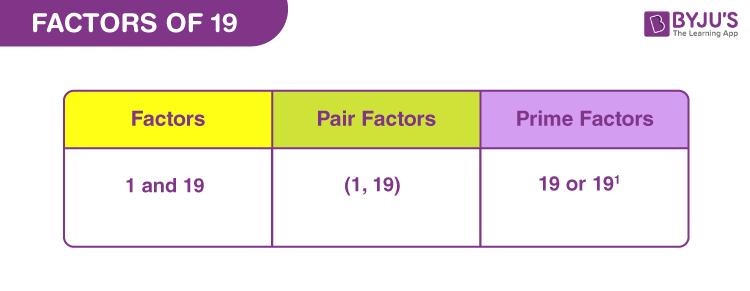# Factors of 19

The factors of 19 are the numbers that divide 19 exactly without leaving any remainder. The factors of 19 can be positive or negative. Similarly, the pair factors of 19 can also be expressed in the positive and negative pairs. For example, the pair factor of 19 is represented by (1, 19) or (-1, 19). If we multiply a pair of negative numbers, such as multiplying -1 and -19, it will result in the original number 19.In this article, we will learn what are the factors of 19, pair factors of 19, and how to find the prime factors of 19 using the prime factorization method.

## What are the Factors of 19?

The numbers that divide 19 completely without leaving a remainder are the factors of 19. In other words, the numbers which are multiplied in pairs, resulting in an original number, are the factors of 19. As number 19 is a prime number, it has only two factors: one and the number itself. Hence, the factors of 19 are 1 and 19, and its negative factors are -1 and -19.

 Factors of 19: 1 and 19. Prime Factorization of 19: 19 or 191.

## Pair Factors of 19

A pair of numbers multiplied together, resulting in the number 19, is called the pair factor of 19. As 19 is a prime number, it has one positive pair factor and one negative pair factor. The positive and negative pair factors of 19 are given below:

Positive Pair Factors of 19:

 Positive Factors of 19 Positive Pair Factor of 19 1 × 19 (1, 19)

Negative Pair Factors of 19:

 Negative Factors of 19 Negative Pair Factor of 19 1 × 19 (-1, -19)

Hence, the positive and negative pair factors of 19 are (1, 19) and (-1, -19), respectively.

## Factors of 19 by Division Method

In the division method, the factors of 19 are found by dividing 19 by different integers. If the integers divide the number 19 exactly, then those integers are the factors of 19. Now, let us discuss how to find the factors of 19 by division method.

• 19/1= 19 (Factor is 1 and Remainder is 0)
• 19/19 = 1 (Factor is 19 and Remainder is 0)

If the numbers other than 1 and 19 divide the number 19, it leaves a remainder of some value. Hence, the factors of 19 are 1 and 19.

## Prime Factorization of 19

In the prime factorization method, 19 is written as the product of its prime factors. Go through the following procedure to find the prime factors of 19.

Take the pair factor of 19, say (1, 19)

Here, both factors 1 and 19 are prime numbers, which cannot be factored further. So, write down the numbers as the product of the prime factors.

Thus, 19 is written as 1×19.

Hence, the prime factorization of 19 is 1×19 or 191.

Note: If a factor is a composite number, split the number into its prime factors and finally write it as the product of prime factors.

 Also, read: Factors of 12 Factors of 15 Factors of 48 Factors of 72

### Solved Examples

Example 1:

Find the common factors of 19 and 18.

Solution:

The factors of 19 are 1 and 19.

The factors of 18 are 1, 2, 3, 6, 9 and 18.

Thus, the common factor of 19 and 18 is 1.

Example 2:

Find the common factors of 19 and 17.

Solution:

Factors of 19 = 1 and 19.

Factors of 17 = 1 and 17.

As both 19 and 17 are prime numbers, the common factor of 19 and 17 is 1 only.

Example 3:

Find the common factors of 19 and 38.

Solution:

The factors of 19 are 1 and 19.

The factors of 38 are 1, 2, 19 and 38.

Hence, the common factors of 19 and 38 are 1 and 19.

### Practice Problems

Solve the following problems:

1. Determine the common factors of 19 and 27.
2. Find the common factors of 19 and 8.
3. Find the common factors of 19 and 76.

## Frequently Asked Questions on Factors of 19

### What are the factors of 19?

The factors of 19 are 1 and 19, as 19 is a prime number.

### What is the prime factorization of 19?

The prime factorization of 19 is 1×19 or 191.

### What are the positive and negative pair factors of 19?

The positive and negative pair factors of 19 are (1, 19) and (-1, -19), respectively.

### Is 8 a factor of 19?

No, 8 is not a factor of 19. If 19 is divisible by 8, it leaves a remainder, and hence 8 is not a factor of 19.

### What is the sum of factors of 19?

The sum of factors of 19 is 20. We know that the factors of 19 are 1 and 19.
Hence, the sum of factors of 19 = 1+19 = 20.